### Conditional Expression

Write and run a program that reads two integers and then uses the conditional expression operator to print either "Multiple" or "Not" according to whether one of the integers is a multiple of the other.

<< Go to Index Page >>

1.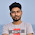#include

main()
{
int a, b;
while(scanf("%d %d", &a, &b)==2)
{
if(a%b==0||b%a==0)
{
printf("Multiple\n");
}
else
{
printf("Not\n");
}
}
return 0;
}

2.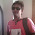Hope you will be continued....

3.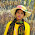#include"stdio.h"
void main()
{
int a,b;
scanf("%d",&a);
scanf("%d",&b);
printf("\nResult is:");
if(a>b)
{
if(a%b==0)
printf("\nMultiple");
else
printf("\nNot Multiple");
}
else if(a<b)
{
if(b%a==0)
printf("\nMultiple");
else
printf("\nNot Multiple");
}
else
printf("\nMultiple");

getch();
}

4.#include

int main()
{
int a, n;
printf("Enter a number: ");
scanf("%d", &n);
for(a = 0; a <= n; a++)
{
if (n == a * a)
{
printf("YES");
return 0;
}
}
printf("NO");
return 0;
}

5.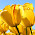Summer 2017
ID#201610042

Answer to the Question No 6:

6.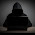https://www.dropbox.com/s/a3hcy9jcu5oycay/check06.cpp?dl=0

7.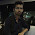ID : 201620195

8.9.10.11.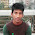ID:201520552

12.ID 201610456
MD USUF MIA

13.14.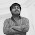Id= 201710494
https://www.dropbox.com/s/o55m0k7gpvbjrmm/prob%206.cpp?dl=0

15.16.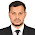17.ID: 201620318

18.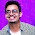19.20.ID:-201610058

21.#include

int main()
{
int age;

scanf("%d",&age);

(age >= 18) ?
printf(" You are eligible to Vote ") :
printf(" You are not eligible to Vote ");

return 0;
}

22.#include

int main()
{
int age;

scanf("%d",&age);

(age >= 18) ?
printf(" You are eligible to Vote ") :
printf(" You are not eligible to Vote ");

return 0;
}

23.#include

int main()
{
int age;

scanf("%d",&age);

(age >= 18) ?
printf(" You are eligible to Vote ") :
printf(" You are not eligible to Vote ");

return 0;
}

24.#include

int main()
{
int age;

scanf("%d",&age);

(age >= 18) ?
printf(" You are eligible to Vote ") :
printf(" You are not eligible to Vote ");

return 0;
}

25.Name- Md.Shariat-ullah
ID No- 201811211

#include
int main()
{
int age;
scanf("%d",&age);
(age>= 18) ?
printf(" You are eligible to Vote ") :
printf(" You are not eligible to Vote ");
return 0;
}

26.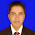Id: 201820244
Batch:58th
section:B

#include
using namespace std;

class check{

public:

int x,y;
void call()
{

if(x%y==0 || y%x==0)
cout<<"Multiple"<<endl;
else
cout<<"Not"<<endl;
}
};

int main()
{
check obj;
obj.call();
}

27.#include
using namespace std;

class check{

public:

int x,y;
void call()
{

if(x%y==0 || y%x==0)
cout<<"Multiple"<<endl;
else
cout<<"Not"<<endl;
}
};

int main()
{
check obj;
obj.call();
}

28.ID#201830546

#include
using namespace std;

class check{

public:

int a,b;
void c()
{
cin>>a>>b;
if(a%b==0 || b%a==0)
cout<<"Multiple"<<endl;
else
cout<<"Not"<<endl;
}
};

int main()
{
check cc;
cc.c();
}

29.ID-201820298
58 BATCH

#include
using namespace std;

class cexp
{
public:
int x,y;
void cexp1()
{
cin>>x>>y;
if(x%y==0||y%x==0)
cout<<"Multiple";
else
cout<<"Not";
}

};
int main()
{
cexp cexp2;
cexp2.cexp1();
}

30.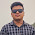Name : Firoj ahmed
Id : 201820222
Batch : 58th

1.

#include
using namespace std;

class myclass
{
public:
int a,b;
myclass()
{
cin >> a >> b;
cout << ( !(a%b) || !(b%a) ? "Multiple" : "Not")<<endl;
}
};

int main()
{
myclass obj;
return 0;
}

31.ID 201820153
BATCH 58TH
SEC B
#include
using namespace std;

class number
{
int a, b;

bool check(void)
{
if (!a||!b)
return false;

if (!(a%b)||!(b%a))
return true;
else
return false;
}
public:

void input (void)
{
cout<<"Enter two integer: ";
cin>>a>>b;
}
void print_status(void)
{
if (check())
cout<<"Multiple"<<endl;
else
cout<<"Not"<<endl;
}
};

int main()
{
number obj;

obj.input();
obj.print_status();

return 0;
}

32.ID. : 201810461
Batch : 57th
//Conditional expression using inheritance//
#include
using namespace std;
class Habibur Rahman
{
public:
int a=5,b=15;

};
class B : public Habibur Rahman
result=a<b ? a : b;
}
cout<<"The result is:"<<result;
}

33.ID. :201810461
Batch :57th
#include
using namespace std;
int main()
{
int num1, num2;
float num3,num4;
cout<<"Enter two integer numbers:" << Result;
cin>>num1>>num2;
cout<<"Result: "<num2) ? end1;
cout <<"Enter two float numbers:" << Result;
cin >>num3>>num4;
cout<<"Result: "<num4) ? end1;
return0;
}
int sum(int a,int b){
return a+b;
}
float sum(float a,flaot b){
return a+b;
}